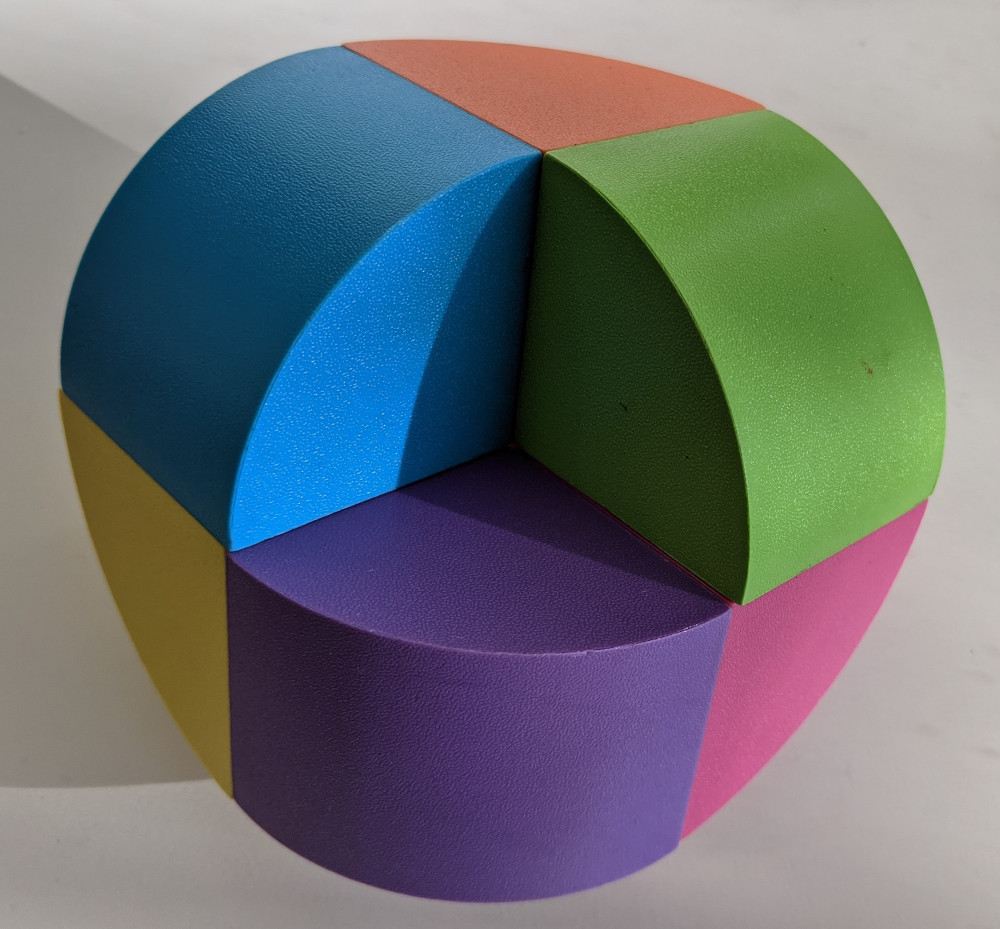# teaching machines

October 5, 2020 by . Filed under madeup, public.

A couple years ago, my wife and I picked up some colorful blocks at a toy store. Each block is a quarter of a cylinder. The square faces of the blocks snap together with help from two magnets inside. There are just enough blocks in the set to make this fascinating shape:I’m struggling to find a name for this shape.

Last week I rewrote my dowel generation algorithm in Madeup, so my subconscious mind informed me that this shape is a dowel. My conscious mind set to modeling it. My first draft just traced out the path from one block to the next using turtle geometry:



moveto(x = 0, y = 0)

move(distance = 1)
yaw(degrees = 90)
move(distance = 1)
pitch(degrees = -90)
move(distance = 1)
yaw(degrees = 90)
move(distance = 1)
pitch(degrees = -90)
move(distance = 1)
yaw(degrees = 90)
move(distance = 1)
pitch(degrees = -90)

dowel(sharpness = 5)

var mupDiv = jQuery('#mup_shape-unrolled');
mupDiv.closest('pre').replaceWith(mupDiv);
document.getElementById('mup_form_shape-unrolled').submit();

moveto(x = 0, y = 0)

move(distance = 1)
yaw(degrees = 90)
move(distance = 1)
pitch(degrees = -90)
move(distance = 1)
yaw(degrees = 90)
move(distance = 1)
pitch(degrees = -90)
move(distance = 1)
yaw(degrees = 90)
move(distance = 1)
pitch(degrees = -90)

dowel(sharpness = 5)



The code makes the repetition visible. I condensed it with this loop:



moveto(x = 0, y = 0)

repeat 3
move(distance = 1)
yaw(degrees = 90)
move(distance = 1)
pitch(degrees = -90)

dowel(sharpness = 5)

var mupDiv = jQuery('#mup_shape');
mupDiv.closest('pre').replaceWith(mupDiv);
document.getElementById('mup_form_shape').submit();

moveto(x = 0, y = 0)

repeat 3
move(distance = 1)
yaw(degrees = 90)
move(distance = 1)
pitch(degrees = -90)

dowel(sharpness = 5)



My version of the shape has a hole in the middle. This is necessary given the way I round out the bends. If I shrink it any further, the bend geometry starts to degenerate. You can see this for yourself by changing the distance of the move commands.